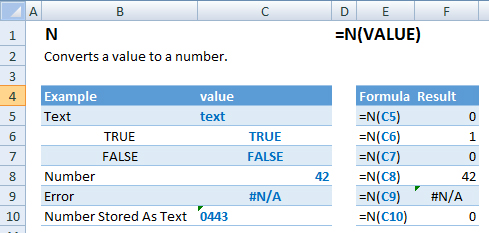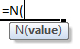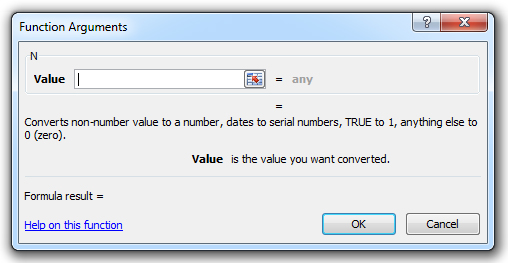### N Function Examples – Excel & Google Sheets

This Excel Tutorial demonstrates how to use the Excel N Function in Excel to convert a value to a number, with formula examples.

## N Function Description:

The N Function Converts a value to a number.

## Formula Examples:Example Formula Result
Text =N(C5) 0
True =N(C6) 1
False =N(C7) 0
Number =N(C8) 42
Error =N(C9) #N/A
Number Stored As Text =N(C10) 0
AutoMacro - VBA Code Generator

## Syntax and Arguments:

The Syntax for the N Formula is:

`=N(VALUE)`

### Function Arguments ( Inputs ):

value – The test value

## Additional Notes

Return to the List of all Functions in Excel

## How to use the N Function in Excel:

To use the AND Excel Worksheet Function, type the following into a cell:
`=AND(`
After entering it in the cell, notice how the AND formula inputs appear below the cell:You will need to enter these inputs into the function. The function inputs are covered in more detail in the next section. However, if you ever need more help with the function, after typing “=N(” into a cell, without leaving the cell, use the shortcut CTRL + A (A for Arguments) to open the “Insert Function Dialog Box” for detailed instructions:For more information about the N Formula visit the
Microsoft Website.

## Excel Practice Worksheet

Practice Excel functions and formulas with our 100% free practice worksheets!

• Automatically Graded Exercises
• Learn Excel, Inside Excel!